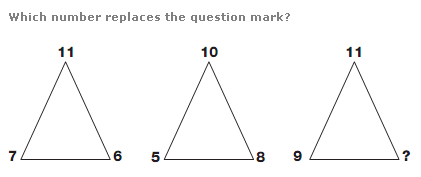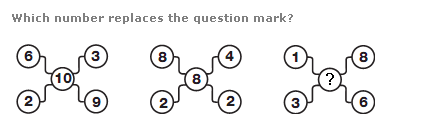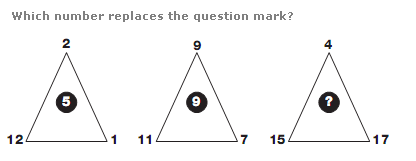# Puzzles - Number puzzles

### Exercise :: Number puzzlesAnswer : 6 Explanation : In each triangle, add together the lower two digits and subtract 2 for the left hand triangle, 3 for the middle and 4 for the right hand triangle, putting the result at the apex of the triangle.Answer : 9 Explanation : Add together the four outer numbers in each diagram. Divide this answer by two to get the middle number.Answer : 12 Explanation : In each triangle, the central value equals the average of the 3 values around the outside.